Custom SearchPRACTICE PROBLEMS: 1. Find, by integration, the area under the curve y=x+4 bounded by the X axis and the lines x=2 and x=7 verify this by a geometric process. 2. Find the area under the curvebounded by the X axis and the lines x = 0 and x = 2 3. Find the area between the curve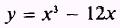and the X axis, from x = -1 to x = 3 ANSWERS: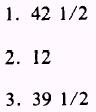SUMMARY The following are the major topics covered in this chapter: 1. Definition of integration: Integration is defined as the inverse of differentiation.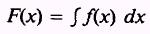where F(x) is the function whose derivative is the function f(x);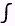is the integral sign; f(x) is the integrand; and dx is the differential. 2. Area under a curve:where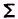(sigma) is the symbol for sum, n is the number of rectangles,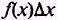is the area of each rectangle, and k is the designation number of each rectangle. Intermediate Value Theorem: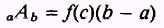where f(c) is the function at an intermediate point between a and b.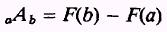where F(b) - F(a) are the integrals of the function of the curve at the values b and a. 3. Indefinite integrals:where C is called a constant of integration, a number which is independent of the variable of integration. Theorem 1. If two functions differ by a constant, they have the same derivative. Theorem 2. If two functions have the same derivative, their difference is a constant. 4. Rules for integration:The integral of a differential of a function is the function plus a constant.A constant may be moved across the integral sign. NOTE: A variable may NOT be moved across the integral sign.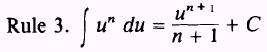The integral of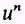du may be obtained by adding 1 to the exponent and then dividing by this new exponent. NOTE: If n is minus 1, this rule is not valid.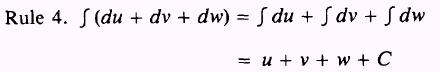The integral of a sum is equal to the sum of the integrals. 5. Definite integrals:where b, the upper limit, and a, the lower limit, are given values. 6. Areas above and below a curve: If the graph of y = f(x), between x = a and x = b, has portions above and portions below the X axis, then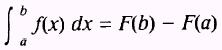is the sum of the absolute values of the positive areas above the X axis and the negative areas below the X axis. ADDITIONAL PRACTICE PROBLEMS Evaluate the following integrals: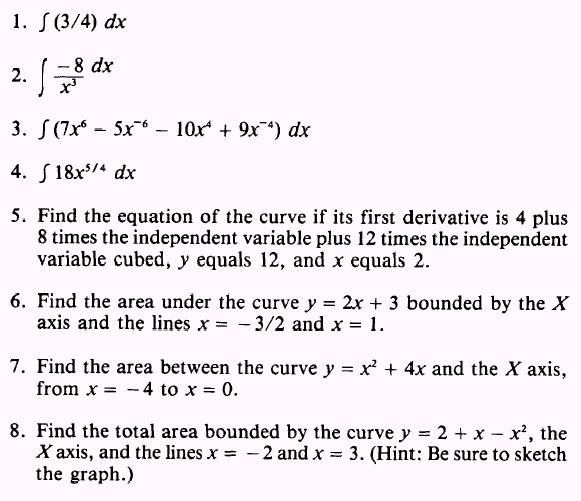ANSWERS TO ADDITIONAL PRACTICE PROBLEMS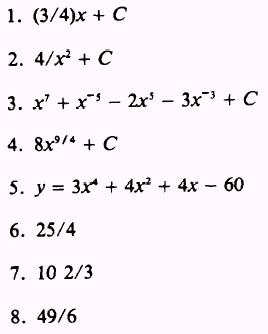Integrated Publishing, Inc. - A (SDVOSB) Service Disabled Veteran Owned Small Business